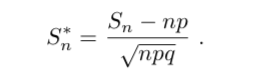#### Elina Azrilyan

3 A true-false examination has 48 questions. June has probability 3/4 of an- swering a question correctly. April just guesses on each question. A passing score is 30 or more correct answers. Compare the probability that June passes the exam with the probability that April passes it.#### June’s Probability

Sn <- 29
n <- 48
p <- 3/4
q <- 1 - p
np <- n*p
sd <- sqrt(n*p*q)

(Sn-np)/sd
##  -2.333333

From Table 9.4, if we interpolate, we would estimate this probability to be .4893

.4893*2
##  0.9786

#### April’s Probability

Sn <- 29
n <- 48
p <- 1/2
q <- 1 - p
np <- n*p
sd <- sqrt(n*p*q)

(Sn-np)/sd
##  1.443376

From Table 9.4, if we interpolate, we would estimate this probability to be

0.5 - .4192
##  0.0808